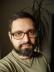## Perl Weekly Challenge 010: Roman Numerals

Write a script to encode/decode Roman numerals.

This has been solved many times, you can easily google a golfed solution shorter than L characters (for example at code-golf.io).

## From Roman

I declared the hash table that binds the corresponding number to each symbol:

``````my %from_roman = (
I => 1,
V => 5,
X => 10,
L => 50,
C => 100,
D => 500,
M => 1000,
);``````

When converting from a Roman numeral, we can’t just add all the numbers corresponding to all the symbols, though, we need to solve the subtractive notation, too. I used a substitution with a regex so solve it:

``````sub from_roman {
my (\$roman) = @_;
my \$n = 0;
while (\$roman =~ s/(I[VXLCDM]|X[LCDM]|C[DM])//) {
my (\$minus, \$plus) = split //, \$1;
\$n += \$from_roman{\$plus} - \$from_roman{\$minus};
}
\$n += \$from_roman{\$_} for split //, \$roman;
return \$n
}``````

## To Roman

Converting the other way round is a bit more complex. The basic hash is pretty simple:

``my %to_roman = reverse %from_roman;``

We can construct a Roman numeral using just this hash, ignoring the subtractive notation.

``````sub to_roman {
my (\$n) = @_;
my \$roman = "";
while (\$n) {
for my \$i (sort { \$b <=> \$a } keys %to_roman) {
while (\$n >= \$i) {
\$n -= \$i;
\$roman .= \$to_roman{\$i};
}
}
}
return \$roman
}``````

We can now again use a substitution to handle the subtractive notation: we’ll just replace each occurrence of IIII by IV, VIIII by IX, etc. This step can be expressed as a hash, again:

``````my %subtractive = (
IIII  => 'IV',
VIIII => 'IX',
XXXX  => 'XL',
LXXXX => 'XC',
CCCC  => 'CD',
DCCCC => 'CM',
);``````

I also tried to express this as an algorithm instead of listing all the possibilities, but I wasn’t happy with the result: the original was definitely more readable.

``````my %subtractive = map {
my \$next5 = \$to_roman{ 5 * \$from_roman{\$_} };
\$_ x 4 => \$_ . \$next5,
\$next5 . \$_ x 4 => \$_ . \$to_roman{ 10 * \$from_roman{\$_} }
}  map \$to_roman{\$_},
grep /10{0,2}\$/, keys %to_roman;``````

To apply the subtractive notation, we need to replace all the keys by their values. The trick is to replace the longer keys first, otherwise VIIII would have been replaced by VIV.

``````my \$subtractive_re = join '|',
sort { length \$b <=> length \$a }
keys %subtractive;``````

And before returning `\$roman` from `to_roman`, let’s just apply all the possible substitutions:

``    \$roman =~ s/(\$subtractive_re)/\$subtractive{\$1}/g;``

To verify the correctness, I copied all the examples from Wikipedia (and maybe added some examples myself):

``````use Test::More;

my %test = (XXXIX     => 39,
CCXLVI    => 246,
DCCLXXXIX => 789,
MMCDXXI   => 2421,
CLX       => 160,
CCVII     => 207,
MIX       => 1009,
MLXVI     => 1066,
MDCCLXXVI => 1776,
MCMIII    => 1903,  # MDCDIII
MCMX      => 1910,
MCMLIV    => 1954,
MCMXCIX   => 1999,  # MIM
MMXIX     => 2019,
MMMCMXCIX => 3_999);

for my \$roman (keys %test) {
is from_roman(\$roman), \$test{\$roman};
}

for my \$roman (keys %test) {
is to_roman(\$test{\$roman}), \$roman;
}``````

Have you noticed the two comments? They introduce alternative ways of writing particular numbers that were actually used by some people or companies in the past. Although our algorithm can’t produce the Roman numerals, it easily converts them correctly to the corresponding numbers:

``````is from_roman('MIM'), 1999;
is from_roman('MDCDIII'), 1903;``````

## Jaro-Winkler Distance

The description of the algorithm in the Wikipedia page was unclear. I tried to implement it, but I couldn’t find answers to my questions in the article. Studying the linked source code didn’t help, as the algorithm was different to the one described. You can check the “Talk” page for a discussion of several unclear parts.

In the end, I turned to CPAN. Text::JaroWinkler to the rescue!

## 1 CommentI blog about Perl.